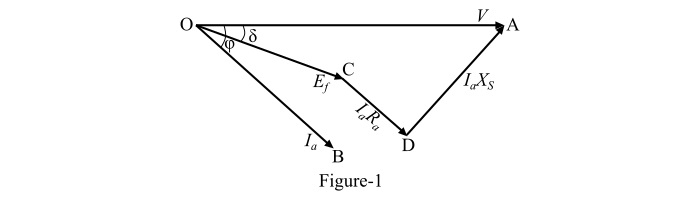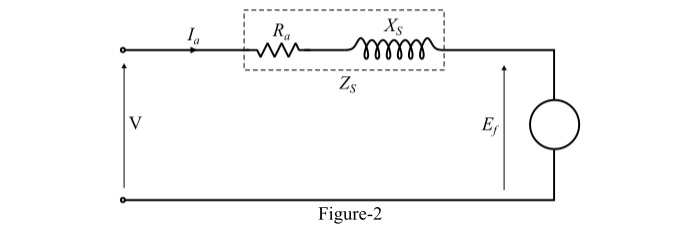# What are the Input Powers of a Synchronous Motor?

The phasor diagram at lagging power factor and the equivalent circuit diagram of a cylindrical synchronous motor are shown in Figure-1 and Figure-2, respectively.The terminal voltage (V) is taken as reference phasor and the excitation voltage (Ef) lags the terminal voltage (V) by an angle δ so that

$$\mathrm{V=V\angle0°\:and\:E_{f}=E_{f}\:\angle-δ}$$

Applying KVL in the loop of equivalent circuit, we get,

$$\mathrm{V=E_{f}+I_{a}Z_{S}\:\:\:\:\:\:...(1)}$$

$$\mathrm{\therefore\:I_{a}=\frac{V-E_{f}}{Z_{S}}\:\:\:\:\:\:...(2)}$$

$$\mathrm{\Longrightarrow\:I_{a}=\frac{V\angle0°-E_{f}\:\angle-δ}{Z_{S}\angleθ_{Z}}=\frac{V}{Z_{S}}\angle-θ_{Z}-\frac{E_{f}}{Z_{S}}\angle-(δ+θ_{Z})}$$

$$\mathrm{\therefore\:I^*_{a}=\frac{V}{Z_{S}}\angleθ_{Z}-\frac{E_{f}}{Z_{S}}\angle(δ+θ_{Z})\:\:\:\:\:\:...(3)}$$

Therefore, the expressions for various input powers to a synchronous motor are given as follows −

## Complex Power Input per Phase to the Synchronous Motor

The complex input power to a synchronous motor is given by,

$$\mathrm{S_{i}=VI^*_{a}=P_{i}+jQ_{i}\:\:\:\:\:\:...(4)}$$

From Eqns.(3) and (4), we get,

$$\mathrm{S_{i}=\frac{V^{2}}{Z_{S}}\angleθ_{Z}-\frac{VE_{f}}{Z_{S}}\angle(δ+θ_{Z})}$$

$$\mathrm{\Longrightarrow\:S_{i}=\left(\frac{V^{2}}{Z_{S}}cosθ_{Z}+j\frac{V^{2}}{Z_{S}}sinθ_{Z}\right)-\left(\frac{VE_{f}}{Z_{S}}cos(δ+θ_{Z})+j\frac{VE_{f}}{Z_{S}}sin(δ+θ_{Z})\right )}$$

$$\mathrm{\therefore\:S_{i}=\left[\frac{V^{2}}{Z_{S}}cosθ_{Z}-\frac{VE_{f}}{Z_{S}}cos(δ+θ_{Z})\right]+j\left[\frac{V^{2}}{Z_{S}}sinθ_{Z}-\frac{VE_{f}}{Z_{S}}sin(δ+θ_{Z})\right]\:\:\:\:\:\:...(5)}$$

## Real Input Power per Phase to the Synchronous Motor

By equating the first term of Equation(5), we get the real power input to the synchronous motor, i.e.,

$$\mathrm{P_{i}=\frac{V^{2}}{Z_{S}}cosθ_{Z}-\frac{VE_{f}}{Z_{S}}cos(δ+θ_{Z})}$$

$$\mathrm{\because\:cosθ_{Z}=\frac{R_{a}}{Z_{S}}}$$

$$\mathrm{\therefore\:P_{i}=\frac{V^{2}}{Z^{2}_{S}}R_{a}-\frac{VE_{f}}{Z_{S}}cos(δ+θ_{Z})\:\:\:\:\:\:...(6)}$$

But,

$$\mathrm{θ_{Z}=90°-α_{Z}}$$

$$\mathrm{cos(δ+θ_{Z})=cos(90°+δ-α_{Z})=-sin(δ-α_{Z})}$$

Therefore, the real power input per phase of the synchronous motor is,

$$\mathrm{P_{i}=\frac{V^{2}}{Z^{2}_{S}}R_{a}+\frac{VE_{f}}{Z_{S}}sin(δ-α_{Z})\:\:\:\:\:\:...(7)}$$

## Reactive Input Power per Phase to the Synchronous Motor

By equating the imaginary part (second term) of Equation (5), we get the values of reactive power input of the synchronous motor, i.e.,

$$\mathrm{Q_{i}=\frac{V^{2}}{Z_{S}}sinθ_{Z}-\frac{VE_{f}}{Z_{S}}sin(δ+θ_{Z})}$$

$$\mathrm{\because\:sinθ_{Z}=\frac{X_{S}}{Z_{S}}}$$

$$\mathrm{\:Q_{i}=\frac{V^{2}}{Z^{2}_{S}}X_{S}-\frac{VE_{f}}{Z_{S}}sin(δ+θ_{Z})\:\:\:\:\:\:...(8)}$$

But,

$$\mathrm{θ_{Z}=90°-α_{Z}}$$

$$\mathrm{sin(δ+θ_{Z})=sin(90°+δ-α_{Z})=cos(δ-α_{Z})}$$

Thus, the reactive power input of the synchronous motor is,

$$\mathrm{Q_{i}=\frac{V^{2}}{Z^{2}_{S}}X_{S}-\frac{VE_{f}}{Z_{S}}cos(δ-α_{Z})\:\:\:\:\:\:...(9)}$$